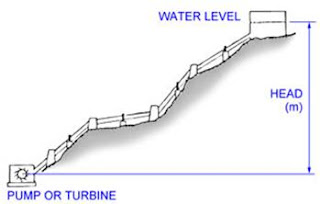# Hydrodynamics

Introduction
“The study of fluids in motion is called hydrodynamics”.
The study is based upon the physical conservation laws of mass, momentum, and energy. The mathematical statements of these laws may be written in either integral or differential form. The integral form is useful for large-scale analyses and provides answers that are sometimes very good and sometimes not, but that are always useful, particularly for engineering applications. The differential form of the equations is used for small-scale analyses. In principle, the differential forms may be used for any problem, but exact solutions can be found only for a small number of specialized flows. Applications of hydrodynamics include the study of closed-conduit and open-channel flow, and the calculation of forces on submerged bodies.

Energy of A Flowing Fluid
“The ability to do work is called energy”.
Major types of energies frequently discussed in hydraulics are as follows. In hydraulics, energy is also referred to as “head”.Types of Energy
1. Pressure Head/Pressure energy is the height to which a fluid will rise because of its pressure. In other words, the energy possessed by a fluid due to its pressure.
hp = p /  g
p = pressure (Pa)
= density (kg/m3)
g = gravitational acceleration(9.81 m/s2)Flowing Water (Kinetic Energy)
2. Velocity Head/Kinetic energy is the height to which a fluid will rise because of its velocity.The energy possessed by a fluid doe to its velocity.
hvv2 / 2g
v= average velocity of the fluid (m/s)
g = gravitational acceleration (9.81 m/s2)Potential Head
3 . Potential Head/Potential energy is the height of the fluid. (above a certain datum).The energy possessed by a fluid due to its elevation.
h=Z
H = h + hv + hp
H = Z + 2/ 2g + p /  g
[blogger]

Engineeersdaily

Name

Email *

Message *